Get instant live expert help with Excel or Google Sheets“My Excelchat expert helped me in less than 20 minutes, saving me what would have been 5 hours of work!”

#### Post your problem and you’ll get expert help in seconds.

Your message must be at least 40 characters
Our professional experts are available now. Your privacy is guaranteed.

# Excel DATEVALUE Function

Excel has a unique way of storing date and time through serial numbers, making it possible to perform mathematical operations and conversions.  Dates that are represented as text values can be easily converted to serial numbers by using the DATEVALUE function in Excel.  This tutorial will assist all levels of Excel users in the usage and syntax of DATEVALUE function.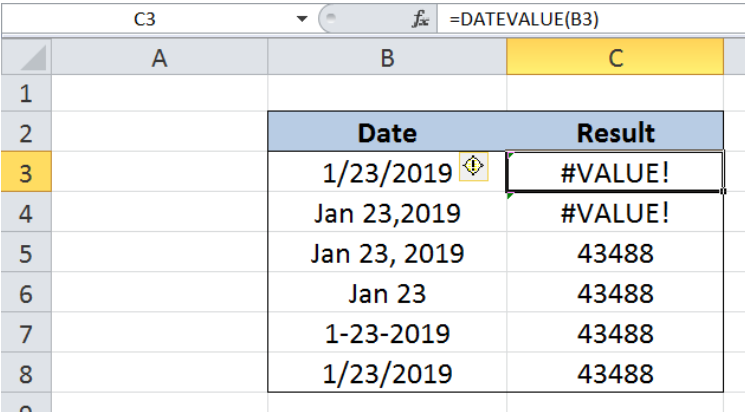Figure 1.  Final result: Excel DATEVALUE function

## Syntax of the DATEVALUE Function

DATEVALUE function converts a date stored as text into a serial number, where the value 1 represents the date Jan 1, 1900 or 1/1/1900

`=DATEVALUE(date_text)`

• Date_text – a text string representing a date in Excel; can be a date enclosed in quotation marks or a cell reference
• If the year portion in the date is omitted, the current year is used by the function
• The date must be between January 1, 1900 and December 31, 9999, otherwise the DATEVALUE function returns the error #VALUE!
• If the date_text argument includes a time value, the function only returns the value for date, ignoring the time

## Setting up our Data

Here we have a template containing two columns: Date (column B) and Result (column C).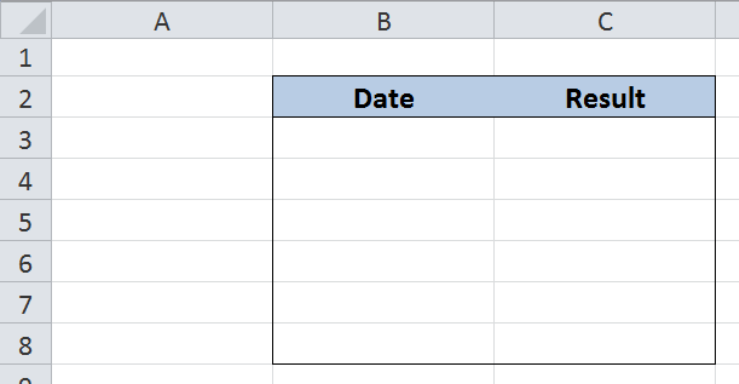Figure 2.  Sample template for Excel DATEVALUE function

To set up our data, enter the following values in cells B3 to B8:

In cell B3, enter the value: 1/23/2019

B4: ‘Jan 23,2019

B5: ‘Jan 23, 2019

B6: ‘Jan 23

B7: ‘1-23-2019

B8: ‘1/23/2019

We want to convert the date “January 23, 2019” into a serial number, but by using different formats.  The results will be recorded in column C.

Note that the value in B3 is inputted in date format, while the rest of the values are inputted as text by using an apostrophe ’ at that start of the text string.  It is important to enter the values exactly as specified above in order to have a better understanding of the DATEVALUE function. After inputting the data in column B,  our table looks like this: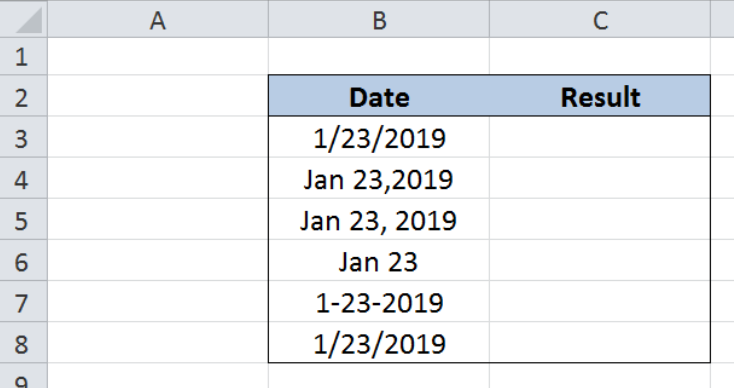Figure 3.  Sample data to convert dates into serial numbers

## Convert dates into serial numbers

We want to convert the date “January 23, 2019” into a serial number by using the DATEVALUE function.  Let us follow these steps:

Step 1.  Select cell C3

Step 2.  Enter the formula: `=``DATEVALUE(B3)`

Step 3.  Press ENTER

Step 4:  Copy the formula in cell C3 to cells C4:C8 by clicking the “+” icon at the bottom-right corner of cell C3 and dragging it down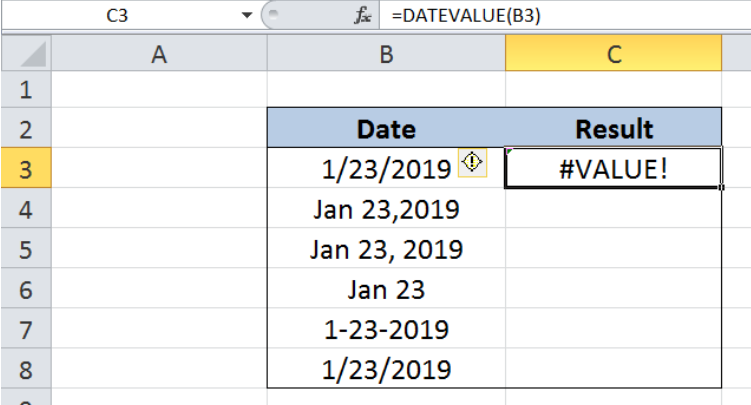Figure 4.  Entering the formula to convert date into serial number using the DATEVALUE function

As discussed earlier, the DATEVALUE function only converts dates in text format.  Cell C3 returns an error value #VALUE! because the date is not entered as text.

Although the value in cell B4 is inputted as text, “Jan 23,2019” is not a valid date because there is no space before the year portion.  As a result, the function also returns a #VALUE! error in cell C4.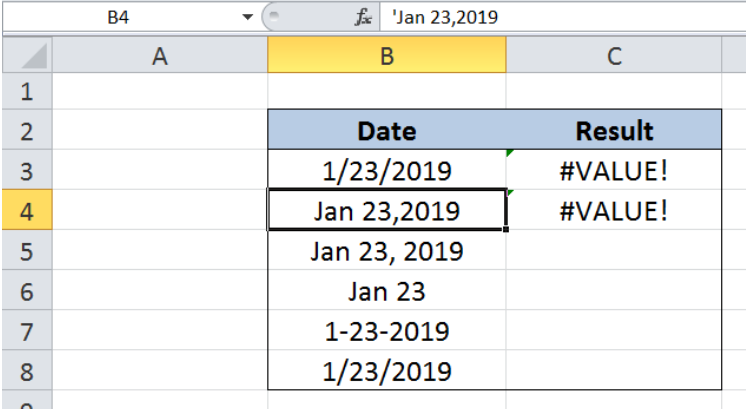Figure 5.  DATEVALUE function returning a #VALUE! error

Cells B5 to B8 contain valid dates in text format.  Hence, the function works properly and returns the value “43488” in cells C5 to C8.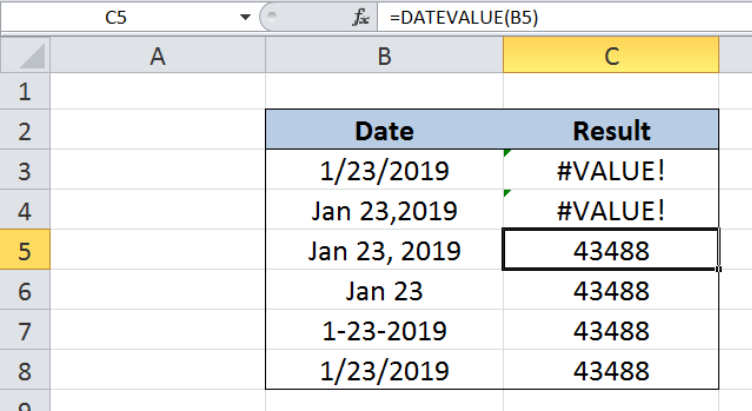Figure 6.  Output: Excel DATEVALUE function

## Note

The date can also be inputted directly into the DATEVALUE function by enclosing it in quotation marks.  Enter this formula in cell C3:

`=DATEVALUE("1/23/2019")`

The result in cell C3 is “43488”, which is the same as in the previous examples.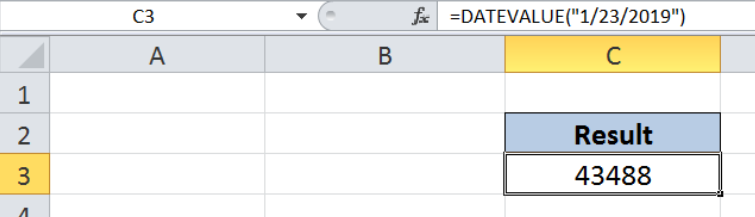Figure 7.  Hardcoding the date into the DATEVALUE function

Most of the time, the problem you will need to solve will be more complex than a simple application of a formula or function. If you want to save hours of research and frustration, try our live Excelchat service! Our Excel Experts are available 24/7 to answer any Excel question you may have. We guarantee a connection within 30 seconds and a customized solution within 20 minutes.

### Did this post not answer your question? Get a solution from connecting with the expert.Another blog reader asked this question today on Excelchat:
Solution examplesI need a formula that has these 2 rules. If letter was rec'd between 1st and 15th = 1st of the month following letter rec'd date. If letter was rec'd between 16thst and end of the month= 1st of the 2nd month following letter rec'd date.
Solved by V. F. in 20 minsI need a column to total (Hours)(85) + (Travel)(.55) per line
Solved by F. Q. in 20 minsTrying to figure out what is wrong with my formula in Oct-ZD tab in column AY and BG.
Solved by O. Q. in 8 minsSo I have used vlookup to find the price of a product on a different sheet and then I have tried to times that by a quantity. e.g. =vlookup(d4,table35,2,false)*e4 This is the formula I typed in which worked for another list I did but for some reason it's not working now even though everything is practically the same, apart from the contents. Could you help?
Solved by B. U. in 60 minsI have a column containing date and time data together and i need to isolate the time data. When I tried to use text to column, it changed all the times to AM. There is an extremely large amount of data - over 550,000 rows so manually changing it won't work. The file is too large to attach.
Solved by S. E. in 40 mins## Subscribe to Excelchat.coAnother blog reader asked this question today on Excelchat: